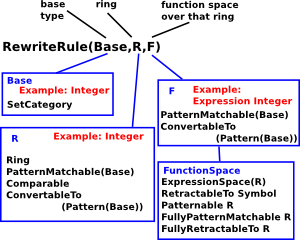To define rules we need a base type and expressions defined in that type. The base type and the expressions require the following:

 Rules must be defined in terms of a category with: FunctionSpace(R) PatternMatchable(Base) ConvertibleTo(Pattern(Base)) in turn FunctionSpace requires: ExpressionSpace(R) RetractableTo Symbol Patternable R FullyPatternMatchable R FullyRetractableTo RThat is, the left and right hand side of the equation must extend these.

For example: Expression(R) extends FunctionSpace(R).

### Interpreter Syntax for Rules

The interpreter seems to have a special syntax for entering rules:

 ```(1) -> R1 := rule log(x) + log(y) == log(x*y) (1) log(y) + log(x) + %B == log(x y) + %B Type: RewriteRule(Integer,Integer,Expression(Integer))```

The simplest form of this special syntax is something like:

'rule' expression '==' expression

but there are more complicated versions like:

'rule' var | predicate var '==' expression

Multiple rules can be entered to a ruleset by using indentation.

I tried doing the same thing, without using this special syntax, I could not get this to work:

 ```(3) -> E1 := log(x) + log(y) (3) log(y) + log(x) Type: Expression(Integer) (4) -> E2 := log(x*y) (4) log(x y) Type: Expression(Integer) (5) -> RR := RewriteRule(Integer,Integer,Expression(Integer)) (5) RewriteRule(Integer,Integer,Expression(Integer)) Type: Type (6) -> R1 := rule(E1,E2)\$RR Line 1: R1 := rule(E1,E2)\$RR .............A Error A: syntax error at top level Error A: Improper syntax. 2 error(s) parsing ```

Why does that not work? I would have thought it would work according to this:

 ```(2) -> )show RewriteRule RewriteRule(Base: SetCategory,R: Join(Ring,PatternMatchable(Base),Comparable, ConvertibleTo(Pattern(Base))),F: Join(FunctionSpace(R),PatternMatchable(Base), ConvertibleTo(Pattern(Base)))) is a domain constructor Abbreviation for RewriteRule is RULE This constructor is exposed in this frame. ------------------------------- Operations -------------------------------- ?=? : (%,%) -> Boolean coerce : Equation(F) -> % coerce : % -> OutputForm elt : (%,F,PositiveInteger) -> F ?.? : (%,F) -> F hash : % -> SingleInteger latex : % -> String lhs : % -> F pattern : % -> Pattern(Base) retract : % -> Equation(F) rhs : % -> F rule : (F,F,List(Symbol)) -> % rule : (F,F) -> % ?~=? : (%,%) -> Boolean hashUpdate! : (HashState,%) -> HashState quotedOperators : % -> List(Symbol) retractIfCan : % -> Union(Equation(F),"failed") suchThat : (%,List(Symbol),(List(F) -> Boolean)) -> % ```

This appears to work, but I don't think it does because E1 and E2 are taken as, variables in the rule, rather than expressions?

 ```(6) -> R1 := rule E1 == E2 (6) E1 == E2 Type: RewriteRule(Integer,Integer,Expression(Integer))```

### Applying the Rules

This is not what I expected:

 ```(7) -> E3 := log(sin(x))+log(y) (7) log(sin(x)) + log(y) Type: Expression(Integer) (8) -> R1 E3 (8) E2 Type: Expression(Integer) (9) -> R1 log(sin(x))+log(y) (9) log(y) + E2 Type: Expression(Integer)```

 ```(6) -> )di op rule There are 2 exposed functions called rule :  (D1,D1,List(Symbol)) -> RewriteRule(D3,D4,D1) from RewriteRule(D3,D4,D1) if D3 has SETCAT and D4 has Join(Ring,PatternMatchable(D3), Comparable,ConvertibleTo(Pattern(D3))) and D1 has Join( FunctionSpace(D4),PatternMatchable(D3),ConvertibleTo( Pattern(D3)))  (D1,D1) -> RewriteRule(D2,D3,D1) from RewriteRule(D2,D3,D1) if D2 has SETCAT and D3 has Join(Ring,PatternMatchable(D2), Comparable,ConvertibleTo(Pattern(D2))) and D1 has Join( FunctionSpace(D3),PatternMatchable(D2),ConvertibleTo( Pattern(D2))) ```

see:

 ```(1) -> PMR := PatternMatchResult(Integer, Symbol) (1) PatternMatchResult(Integer,Symbol) Type: Type (2) -> P := Pattern(Integer) (2) Pattern(Integer) Type: Type (3) -> p := (1\$Integer) :: P (3) 1 Type: Pattern(Integer) (4) -> pmr := new()\$PMR (4) [] Type: PatternMatchResult(Integer,Symbol) (5) -> patternMatch("*"::Symbol,p,pmr)\$Symbol (5) Does not match Type: PatternMatchResult(Integer,Symbol) ```